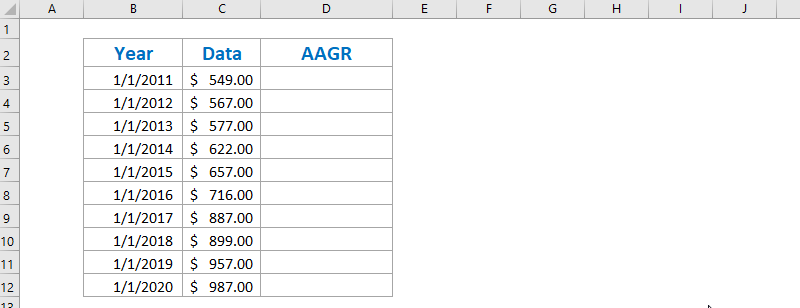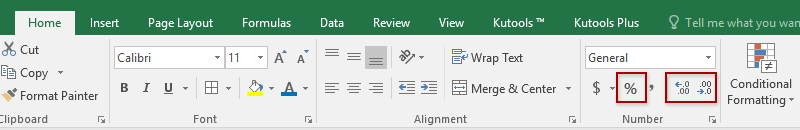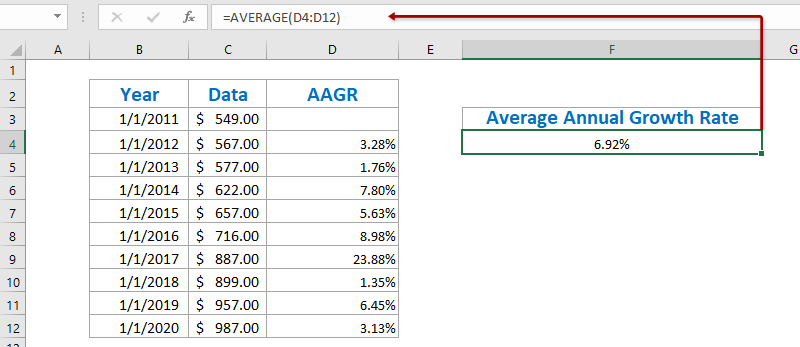I have a last year sale and some currant year sale so I want calculate growth rate in F3 Cell number and I have used this formula =SUM(D3:D4)/SUM(C3:C4)-1 to calculate the growth rate.

Something like this:If I add some Sale in Current year then I drag down sum formula range in growth rate formula:I want automatic Calculate growth rate if I add new value in Current year and then automatic collect Sale from last year in same currant year sale and calculate growth rate.

Note: Growth rate will be Calculate day by day...Apr 1 in Database 30 views

## 1 answer to this question.

In order to get the Average Annual Growth Rate in Excel, we must first calculate the annual growth rates for each year using the formula = (Ending Value - Beginning Value) / Beginning Value, and then average them.

Besides the original table, enter the below formula into the blank Cell C3 and, and then drag the Fill Handle to the Range C3:C11.

=(C4-C3)/C32. On the Home tab, select the Range D4:D12, then click the Percent Style button to adjust the decimal places by clicking the Increase Decimal or Decrease Decimal buttons.3. Enter the formula below into Cell F4 and click the Enter key to average all annual growth rates.

=AVERAGE(D4:D12)answered Apr 4 by
• 8,820 points

## How can I calculate deciles with a range of 12,000 cells in excel?

1. Enter the following formula in cell ...READ MORE

## How to Calculate DATEDIF formula in Excel?

Simply divide one date by the other. ...READ MORE

## How to calculate student pass percentage in excel

Percentage formula in Excel In cell D2, type ...READ MORE

## Group by Sum in Excel

It is very easy and you can ...READ MORE

## Calculate Birthdate from an age using y,m,d in Excel

Hi To Calculate the date, we can ...READ MORE

## Convert Rows to Columns with values in Excel using custom format

1 I having a Excel sheet with 1 ...READ MORE

## IF - ELSE IF - ELSE Structure in Excel

In this case, you can use nested ...READ MORE